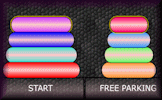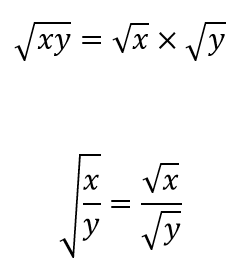Surds - Level 1

A self-marking exercise on calculating, simplifying and manipulating surds (also known as radicals).

Level 1Level 2Level 3Level 4Level 5Level 6Level 7Exam-StyleDescriptionHelp

Without using a calculator simplify the following. You can use the √ button to insert the radical symbol. You can earn a trophy if you get at least 9 questions correct and you do this activity online.

 $$\sqrt{9}$$$$\sqrt{18}$$$$\sqrt{48}$$$$\sqrt{43}$$$$\sqrt{45}$$$$\sqrt{384}$$$$\sqrt{63}$$$$\sqrt{66}$$$$\sqrt{729}$$$$\sqrt{1210}$$$$\sqrt{396}$$$$\sqrt{215}$$Check

This is Surds level 1. You can also try:
Level 2 Level 3 Level 4 Level 5 Level 6 Level 7

Instructions

Try your best to answer the questions above. Type your answers into the boxes provided leaving no spaces. As you work through the exercise regularly click the "check" button. If you have any wrong answers, do your best to do corrections but if there is anything you don't understand, please ask your teacher for help.

When you have got all of the questions correct you may want to print out this page and paste it into your exercise book. If you keep your work in an ePortfolio you could take a screen shot of your answers and paste that into your Maths file.

Transum.org

This web site contains over a thousand free mathematical activities for teachers and pupils. Click here to go to the main page which links to all of the resources available.

Please contact me if you have any suggestions or questions.More Activities:

Mathematicians are not the people who find Maths easy; they are the people who enjoy how mystifying, puzzling and hard it is. Are you a mathematician?

Comment recorded on the 9 May 'Starter of the Day' page by Liz, Kuwait:

"I would like to thank you for the excellent resources which I used every day. My students would often turn up early to tackle the starter of the day as there were stamps for the first 5 finishers. We also had a lot of fun with the fun maths. All in all your resources provoked discussion and the students had a lot of fun."

Comment recorded on the 24 May 'Starter of the Day' page by Ruth Seward, Hagley Park Sports College:

"Find the starters wonderful; students enjoy them and often want to use the idea generated by the starter in other parts of the lesson. Keep up the good work"

Tower of HanoiMove the pieces of the tower from one place to another in the minimum number of moves. This puzzle was invented in 1883 but is still as captivating today as it was all those years ago.

There are answers to this exercise but they are available in this space to teachers, tutors and parents who have logged in to their Transum subscription on this computer.

A Transum subscription unlocks the answers to the online exercises, quizzes and puzzles. It also provides the teacher with access to quality external links on each of the Transum Topic pages and the facility to add to the collection themselves.

Subscribers can manage class lists, lesson plans and assessment data in the Class Admin application and have access to reports of the Transum Trophies earned by class members.

If you would like to enjoy ad-free access to the thousands of Transum resources, receive our monthly newsletter, unlock the printable worksheets and see our Maths Lesson Finishers then sign up for a subscription now:

Subscribe

Go Maths

Learning and understanding Mathematics, at every level, requires learner engagement. Mathematics is not a spectator sport. Sometimes traditional teaching fails to actively involve students. One way to address the problem is through the use of interactive activities and this web site provides many of those. The Go Maths page is an alphabetical list of free activities designed for students in Secondary/High school.

Maths Map

Are you looking for something specific? An exercise to supplement the topic you are studying at school at the moment perhaps. Navigate using our Maths Map to find exercises, puzzles and Maths lesson starters grouped by topic.

Teachers

If you found this activity useful don't forget to record it in your scheme of work or learning management system. The short URL, ready to be copied and pasted, is as follows:

Do you have any comments? It is always useful to receive feedback and helps make this free resource even more useful for those learning Mathematics anywhere in the world. Click here to enter your comments.For All:

© Transum Mathematics :: This activity can be found online at:
wwww.Transum.org/go/?Num=3

Description of Levels

Level 1 - Simplifying surds

Level 2 - Simplifying the product of two surds

Level 3 - Simplifying the product of integers and surds

Level 4 - Simplifying the sum of integers and surds

Level 5 - Simplifying fractions containing surds

Level 6 - Rationalising the denominator of a fraction

Level 7 - Miscellaneous questions involving surds

Exam Style questions are in the style of GCSE exam paper questions and worked solutions are available for Transum subscribers.

Don't wait until you have finished the exercise before you click on the 'Check' button. Click it often as you work through the questions to see if you are answering them correctly. You can double-click the 'Check' button to make it float at the bottom of your screen.

Answers to this exercise are available lower down this page when you are logged in to your Transum account. If you don’t yet have a Transum subscription one can be very quickly set up if you are a teacher, tutor or parent.

There are three statements referring to surds in the English National Curriculum:

• Pupils should be taught to calculate exactly with fractions, {surds} and multiples of π ; {simplify surd expressions involving squares [for example √12 = √(4 × 3) = √4 × √3 = 2√3] and rationalise denominators}
• Pupils should be taught to simplify and manipulate algebraic expressions including those involving surds ...
• Pupils should be taught to recognise and use sequences ... and simple geometric progressions (rn where n is an integer, and r is a positive rational number {or a surd})

CloseThe key properties you need are:Examples

$$\sqrt{80} = \sqrt{4\times4\times5} = \sqrt{4}\times\sqrt{4}\times\sqrt{5} = 2\times2\times\sqrt{5} = 4\sqrt{5}$$

$$\sqrt{8}\times\sqrt{12} = \sqrt{4\times2}\times\sqrt{4\times3} = 2\times\sqrt{2}\times2\times\sqrt{3} = 4\sqrt{6}$$

$$(5+\sqrt{7})(5-\sqrt{7}) = 25+5\sqrt{7}-5\sqrt{7}-7 = 25-7 = 18$$

This video is from UKMathsTeacher.

Don't wait until you have finished the exercise before you click on the 'Check' button. Click it often as you work through the questions to see if you are answering them correctly. You can double-click the 'Check' button to make it float at the bottom of your screen.

Answers to this exercise are available lower down this page when you are logged in to your Transum account. If you don’t yet have a Transum subscription one can be very quickly set up if you are a teacher, tutor or parent.

Close